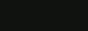# test (1)

NAME
SYNOPSIS
DESCRIPTION
OPTIONS
EXIT STATUS
Source Code

## NAME

test - evaluate expression

## SYNOPSIS

 test expression test [

expression ]

 [ ]

## DESCRIPTION

The test utility evaluates the expression and indicates the result of the evaluation by its exit status.

## OPTIONS

-b pathname

True if pathname resolves to an existing block special file.

-c pathname

True if pathname resolves to an exisiting character special file.

-d pathname

True if pathname resolves to an existing directory.

-e pathname

True if pathname resolves to an existing directory entry.

-f pathname

True if pathname resolves to an existing regular file.

-g pathname

True if pathname resolves to an existing file that has its set-group-ID flag set.

-h pathname

True if pathname resolves to an existing symbolic link.

.L pathname

True if pathname resolves to an existing symbolic link.

-n string

True if the length of string is non-zero.

-p pathname

True if pathname resolves to an existing FIFO.

-r pathname

True if pathname resolves to an existing file for which permission to read the file will be granted.

-S pathname

True if pathname resolves to an existing socket.

-s pathname

True if pathname resolves to an existing file that has a size greater than zero.

-t file_descriptor

True if file_descriptor is open and associated with a terminal.

-u pathname

True if pathname is resolved to an existing file that has its set-user-ID flag set.

-w pathname

True if pathname resolves to an existing file for which permission to write to the file will be granted.

-x pathname

True if pathname resolves to an existing file for which permission to execute the file (or search it, if it is a directory) will be granted.

-z string

True if the length of string is zero.

string

True if the length of string is non-zero.

s1 = s2

True if strings s1 and s2 are identical.

s1 != s2

True if strings s1 and s2 are not identical.

n1 -eq n2

True if the integers n1 and n2 are algebraically equal.

n1 -ne n2

True if the integers n1 and n2 are not algebraically equal.

n1 -gt n2

True if the integer n1 is algebraically greater than the integer n2.

n1 -ge n2

True if the integer n1 is algebraically greater than or equal to the integer n2.

n1 -lt n2

True if the integer n1 is algebraically less than the integer n2.

n1 -le n2

True if the integer n1 is algebraically less than or equal to the integer n2.

expr1 -a expr2

True if both expressions expr1 and expr2 are true.

expr1 -o expr2

True if at least one of the expressions expr1 and expr2 is true.

! expression

True if the expression is false.

( expression )

True if the expression is true.

## EXIT STATUS

0

expression evaluated to true.

1

expression evaluated to false or expression was missing.

>1

An error occured.